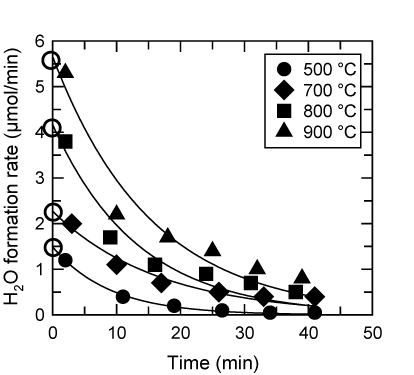wFigure 2: A plot of the H2O formation rate as a function of the reaction time used to determine the initial H2O formation rate. Open circles represent the initial H2O formation rate. At reaction time zero, the initial H2O formation rate was estimated by a first-order rate equation, R=R0 exp(-kt).